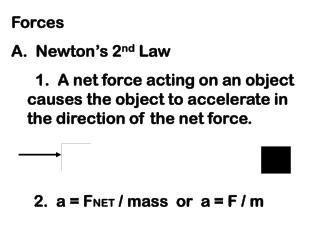DownloadDownload PresentationForces A. Newton’s 2 nd Law 1. A net force acting on an object causes the object to accelerate in the direction

# Forces A. Newton’s 2 nd Law 1. A net force acting on an object causes the object to accelerate in the direction

Télécharger la présentation## Forces A. Newton’s 2 nd Law 1. A net force acting on an object causes the object to accelerate in the direction

- - - - - - - - - - - - - - - - - - - - - - - - - - - E N D - - - - - - - - - - - - - - - - - - - - - - - - - - -
##### Presentation Transcript

1. Forces A. Newton’s 2nd Law 1. A net force acting on an object causes the object to accelerate in the direction of the net force. 2. a = FNET / mass or a = F / m

2. B. Friction 1. Force that opposes motion between two surfaces in contact. 2. Amount depends on: a. Kinds of surfaces in contact. b. Amount of force pressing surfaces together. Something that weighs more will have greater friction.

3. 3. Friction is caused by microwelds 4. Types of friction: a. Static (usually the greatest) b. Sliding c. Rolling (usually the least)

4. C. Air resistance (drag force) 1. Force that opposes motion of objects through air 2. Pushes up on falling objects 3. Affected by object’s speed, size, shape

5. 4. Without drag force, all objects fall at the same rate 5. Terminal velocity is the max speed at which an object can fall

6. D. Gravity 1. Attraction between objects 2. Weakest force in universe 3. Farthest range 4. Directly proportional to the masses of the objects 5. Inversely proportional to the squares of the distance between

7. E. Gravitational Acceleration 1. g = 9.8 m/s/s on Earth 2. FWEIGHT = m x g 3. All objects fall with the same g 4. Weight is NOT the same as mass

8. F. Free Fall (Weightlessness) 1. As long as an object is free falling, nothing exerts an upward force 2. With no upward force, FW = 0 N

9. G. Projectile and Circular Motion 1. Projectile motion a. Follow a curved path b. Two types of motion are independent of one another: 1) Horizontal (based on initial velocity and inertia) 2) Vertical (based on gravity)

10. c. An object launched horizontally will land at the same time as an object simply dropped from the same height 2. Circular Motion a. Objects moving in circular paths accelerate toward the center b. Centripetal acceleration c. Centripetal force (FC = m x aC)

11. d. Centrifugal force is imaginary e. Weightlessness in orbit exists because objects are constantly falling toward Earth, but have enough forward velocity to keep them in orbit